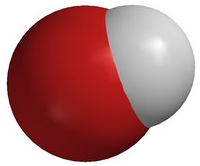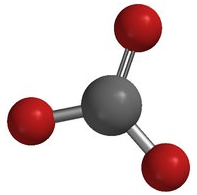# 2.5: Ion Formation

•• Contributed by Allison Soult
• Senior Lecturer (Chemistry) at University of Kentucky

Learning Outcomes

• Define ion, cation, and anion.
• Recognize characteristics of monatomic and polyatomic ions.
• Explain how and why cations and anions are formed.
• Determine the number of subatomic particles in an ion.
• Predict the charge on ions formed from representative elements.

In many parts of the country, including parts of Kentucky, the water contains high concentrations of minerals that stain clothes, build up deposits on bathtubs and water heaters, and create problems with soap foaming properly. This problem is caused by what is called "hard water". The water contains excessive amounts of cations such as iron and calcium.

## Ions

Ions are charged substances that have formed through the gain or loss of electrons. Cations form from the loss of electrons and have a positive charge while anions form through the gain of electrons and have a negative charge.

### Cation Formation

Cations are the positive ions formed by the loss of one or more electrons. The most commonly formed cations of the representative elements are those that involve the loss of all of the valence electrons. Consider the alkali metal sodium $$\left( \ce{Na} \right)$$. It has one valence electron in the $$n=3$$ energy level. Upon losing that electron, the sodiu ion now has an octet of electrons from the second energy level and a charge of $$1+$$.

The electron arrangement of the sodium ion is now the same as that of the noble gas neon. Consider a similar process with magnesium and aluminum. In this case, the magnesium atom loses its two valence electrons in order to achieve the same arrangement as the noble gas neon and a charge of $$2+$$. The aluminum atom loses its three valence electrons to have the same electron arrangement as neon and a charge of $$3+$$. For representative elements under typical conditions, three electrons is usually the maximum number that will be los. Representative elements will not lose electrons beyond their valence because they would have to "break" the octet of the previous energy level which provides stability to the ion.

### Anions

Anions are the negative ions formed from the gain of one or more electrons. When nonmetal atoms gain elections, they often do so until their outermost principal energy level achieves an octet. For fluorine, which has an electron arrangement of (2, 7), it only needs to gain one electron to have the same electron arrangement as neon. Forming an octet (eight electrons in the outer shell) provides stability to the atom. Fluorine will gain one electron and have a charge of $$1-$$. The electron arrangement of the fluoride ion (2, 8) will also change to reflect the gain of an electron.

Oxygen has an electron arrangement of (2, 6) and needs to gain two electrons to fill the $$n=2$$ energy level and achieve an octet of electrons in the outermost shell. The oxide ion will have a charge of $$2-$$ as a result of gaining two electrons. Under typical conditions, three electrons is the maximum that will be gained in the formation of anions.

### Subatomic Particles in an Ion

Since ions form from the gain or loss of electrons, we can also look at the number of subatomic particles (protons, neutrons, and electrons) found in an ion. Remember that the number of protons determines the identity of the element and will not change in a chemical process.

Example $$\PageIndex{1}$$

How many protons, neutrons, and electrons in a single oxide $$\left( \ce{O^{2-}} \right)$$ ion?

Solution

Oxygen has the atomic number 8 so both the atom and the ion will have 8 protons. The average atomic mass of oxygen is 16. Therefore, there will be 8 neutrons $$\left( \text{atomic mass} - \text{atomic number} = \text{neutrons} \right)$$. A neutral oxygen atom would have 8 electrons. However, the anion has gained two electrons so $$\ce{O^{2-}}$$ has 10 electrons.

We can also use information about the subatomic particles to determine the identity of an ion.

Example $$\PageIndex{2}$$

An ion with a $$2+$$ charge has 18 electrons. Determine the identity of the ion.

Solution

If an ion has a $$2+$$ charge then it must have lost electrons to form the cation. If the ion has 18 electrons and the atom lost 2 to form the ion, then the neutral atom contained 20 electrons. Since it was neutral, it must also have had 20 protons. Therefore the element is calcium.

### Polyatomic Ions

A polyatomic ion is an ion composed of two or more atoms that have a charge as a group (poly = many). The ammonium ion (see figure below) consists of one nitrogen atom and four hydrogen atoms. Together, they comprise a single ion with a $$1+$$ charge and a formula of $$\ce{NH_4^+}$$. The hydroxide ion (see figure below) contains one hydrogen atom and one oxygen atom with an overall charge of $$1-$$. The carbonate ion (see figure below) consists of one carbon atom and three oxygen atoms and carries an overall charge of $$2-$$. The formula of the carbonate ion is $$\ce{CO_3^{2-}}$$. The atoms of a polyatomic ion are tightly bonded together and so the entire ion behaves as a single unit. The figures below show several examples.Figure $$\PageIndex{1}$$: The ammonium ion $$\left( \ce{NH_4^+} \right)$$ is a nitrogen atom (blue) bonded to four hydrogen atoms (white).Figure $$\PageIndex{2}$$: The hydroxide ion $$\left( \ce{OH^-} \right)$$ is an oxygen atom (red) bonded to a hydrogen atom.Figure $$\PageIndex{3}$$: The carbonate ion $$\left( \ce{CO_3^{2-}} \right)$$ is a carbon atom (black) bonded to three oxygen atoms.

The table below lists a number of polyatomic ions by name and by structure. The heading for each column indicates the charge on the polyatomic ions in that group. Note that the vast majority of the ions listed are anions - there are very few polyatomic cations.

$$1-$$ $$2-$$ $$3-$$ $$1+$$
Table $$\PageIndex{1}$$: Common Polyatomic Ions
acetate, $$\ce{CH_3COO^-}$$ carbonate, $$\ce{CO_3^{2-}}$$ arsenate, $$\ce{AsO_3^{3-}}$$ ammonium, $$\ce{NH_4^+}$$
bromate, $$\ce{BrO_3^-}$$ chromate, $$\ce{CrO_4^{2-}}$$ phosphite, $$\ce{PO_3^{3-}}$$
chlorate, $$\ce{ClO_3^-}$$ dichromate, $$\ce{Cr_2O_7^{2-}}$$ phosphate, $$\ce{PO_4^{3-}}$$
chlorite, $$\ce{ClO_2^-}$$ hydrogen phosphate, $$\ce{HPO_4^{2-}}$$
cyanide, $$\ce{CN^-}$$ oxalate, $$\ce{C_2O_4^{2-}}$$
dihydrogen phosphate, $$\ce{H_2PO_4^-}$$ peroxide, $$\ce{O_2^{2-}}$$
hydrogen carbonate, $$\ce{HCO_3^-}$$ silicate, $$\ce{SiO_3^{2-}}$$
hydrogen sulfate, $$\ce{HSO_4^-}$$ sulfate, $$\ce{SO_4^{2-}}$$
hydrogen sulfide, $$\ce{HS^-}$$ sulfite, $$\ce{SO_3^{2-}}$$
hydroxide, $$\ce{OH^-}$$
hypochlorite, $$\ce{ClO^-}$$
nitrate, $$\ce{NO_3^-}$$
nitrite, $$\ce{NO_2^-}$$
perchlorate, $$\ce{ClO_4^-}$$
permanganate, $$\ce{MnO_4^-}$$

The vast majority of polyatomic ions are anions, many of which end in -ate or -ite. Notice that in some cases such as nitrate $$\left( \ce{NO_3^-} \right)$$ and nitrite $$\left( \ce{NO_2^-} \right)$$, there are multiple anions that consist of the same two elements. In these cases, the difference between the ions is the number of oxygen atoms present, while the overall charge is the same. As a class, these are called oxyanions. When there are two oxyanions for a particular element, the one with the greater number of oxygen atoms gets the -ate suffix, while the one with the fewer number of oxygen atoms gets the -ite suffix. The four oxyanions of chlorine are shown below, which also includes the use of the prefixes hypo- and per-.

• $$\ce{ClO^-}$$, hypochlorite
• $$\ce{ClO_2^-}$$, chlorite
• $$\ce{ClO_3^-}$$, chlorate
• $$\ce{ClO_4^-}$$, perchlorate"Drink you milk. It's good for your bones." We're told this from early childhood, and with good reason. Milk contains a good supply of calcium, part of the structure of bone. However, there are two other ionic components of hydroxyapatite, the mineral component. Phosphate ion and hydroxide ion make up the remainder of the inorganic material in bone.

News You Can Use

• Bone is a very complex structure. It is composed of protein (mainly collagen), hydroxyapatite (a calcium-phosphate-hydroxide mixture), some other minerals, and contains $$10$$-$$20\%$$ water. The calcium/phosphate ratios are not stoichiometric, but vary somewhat from one portion of bone to the next.
• Bones are very strong but will break under enough stress. Regular exercise and proper nutrition help to increase bone strength. Watch a video about bone structure at http://www.youtube.com/watch?v=d9owEvYdouk
• Nitrate is an anion with a complex bonding structure. Major sources for this ion in drinking water are runoff from fertilizer, septic tank leakage, sewage, and natural deposits. High concentrations of nitrates represent a significant health hazard, especially to infants. The nitrate in the body is converted to nitrite, which then binds to hemoglobin. This binding decreases the ability of hemoglobin to transport oxygen, thus depriving the cells of the $$\ce{O_2}$$ needed for proper functioning.
• Cyanide production is widespread throughout nature. Forest fires will produce significant amounts of cyanide. Many plants contain cyanide, and it is produced by a number of bacteria, algae, and fungi. Cyanide is used industrially in metal finishing, iron and steel mills, and in organic synthesis processes. This material is also an important component for the refining of precious metals. Formation of a complex between cyanide and gold allows extraction of this metal from a mixture.